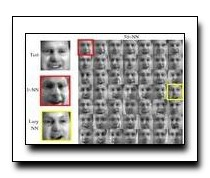Local Distance Functions: A Taxonomy, New Algorithms, and an Evaluation
Deva Ramanan, Simon BakerWe present a taxonomy for local distance functions where most existing algorithms can be regarded as approximations of the geodesic distance defined by a metric tensor. We categorize existing algorithms by how, where, and when they estimate the metric tensor. We also extend the taxonomy along each axis. How: We introduce hybrid algorithms that use a combination of techniques to ameliorate over-fitting. Where: We present an exact polynomial time algorithm to integrate the metric tensor along the lines between the test and training points under the assumption that the metric tensor is piecewise constant. When: We propose an interpolation algorithm where the metric tensor is sampled at a number of references points during the offline phase. The reference points are then interpolated during the online classification phase. We also present a comprehensive evaluation on tasks in face recognition, object recognition, and digit recognition.

### Text Reference

Deva Ramanan and Simon Baker. Local distance functions: a taxonomy, new algorithms, and an evaluation. In IEEE Transactions on Pattern Analysis and Machine Intelligence (PAMI). 2011.

### BibTeX Reference

@inproceedings{RamananB_PAMI_2011,
author = "Ramanan, Deva and Baker, Simon",
booktitle = "IEEE Transactions on Pattern Analysis and Machine Intelligence (PAMI)",
title = "Local Distance Functions: A Taxonomy, New Algorithms, and an Evaluation",
year = "2011",
tag = "object_recognition"
}